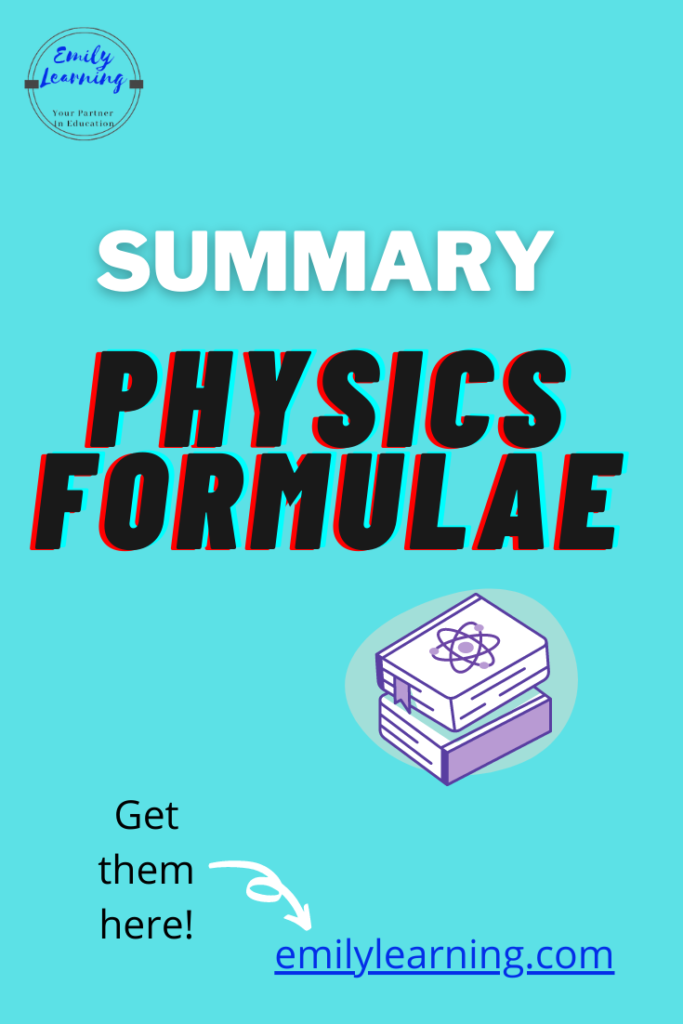# O Level Physics FormulaeIn this post, you will find a list of Formulae for O Level Physics. I have listed the formulae according to the different chapters below.

## O Level Physics Formulae By Chapter

Below are a list of the formulae from various chapters. Click on the link below to go directly to the chapter you are interested in.

Kinematics formulae

Forces formulae

Weight, mass and density formulae

Pressure formulae

Work, Energy and Power Formulae

Thermal Physics Formulae

Waves Formulae

Light Formulae

Sound Formulae

Electricity Formulae

## Kinematics Formulae

average speed = total distance travelled / total time taken

acceleration = (initial velocity – final velocity)/ time

If a = acceleration, u = initial velocity, v = final velocity, s = displacement, t = time, these formulae can be used when acceleration is constant:

• v = u + at
• s = 1/2(u+v)t
• v2 = u2 + 2as
• s = ut + 1/2 at2

## Forces Formulae

If F = resultant force, m = mass and a = acceleration,

F = ma

## Weight, Mass, Density Formulae

If W = weight, m = mass and ρ = density, g = acceleration due to gravity or gravitational field strength, V = volume

W = mg

ρ = m/ V

## Moments Formulae

moment = force x perpendicular distance

## Pressure Formulae

pressure = force/ Area

pressure = hρg

F1/A1 = F2/A2

## Work, Energy and Power Formulae

Work done = Force x distance

where m = mass, g = acceleration due to gravity or gravitational field strength, v = velocity, h = height

Gravitational Potential energy (Ep) = mgh

Kinetic energy (Ek) = 1/2 mv2

Power = energy/ time = work done / time

## Thermal Physics Formulae

where m = mass, c = specific heat capacity, C = heat capacity, l = specific latent heat , Δθ = temperature change, Q = change in thermal energy

Q = mcΔθ

Q = C Δθ

Q = ml

## Waves Formulae

where v = velocity, f = frequency, λ = wavelength, T = period

v = fλ

f = 1/T

## Light Formulae

For reflection, angle of incident = angle of reflection

For refraction where n = refractive index, i = angle of incident in vacuum or air, r = angle of refraction in medium, c is critical angle

n = sin i/ sin r

n = 1 / sin c

## Sound Formulae

speed = distance travelled / time taken

## Electricity Formulae

Q = It

V = W/ Q

R = ρl/A

V= IR

P = VI = I2R = V2/R = energy/ t = work done/ t

Energy = Power x t = VIt = I2Rt = (V2/R)t

#### For series circuits:

Current is the same everywhere in a series circuit

Total potential difference = sum of potential difference across each component

Total resistance = sum of resistance of each component

If we make use of this example of a series circuit below:

#### Relationship between V, I and R in series circuit

For potential difference (p.d.)

V= V1 + V2

V1 = [R1/ (R1+ R2) ]V

V2 = [R2/ (R1+ R2) ]V

For current:

I = I1 = I2

For resistance:

Rtotal = R1 + R2

#### For parallel circuits:

To find total current, we add them up.

When parallel, potential difference is the same

1/ (Total resistance) = 1/R1 + 1/ R2 +…

If we make use of this example of a parallel circuit below:

#### Relationship between V, I and R in parallel circuit

For potential difference (p.d.)

V= V1 = V2

For current:

I = I1 + I2

For resistance:

1/Rtotal = 1/R1 + 1/R2

## Electromagnetic Induction

For 100% efficient transformers:

IpVp = IsVs

Ns/Np = Vs/Vp

where

Ns = number of turns in secondary coil

Vs = potential difference across secondary coil

Is = current in secondary coil

Np = number of turns in primary coil

Vp = potential difference across primary coil

Ip = current in primary coil

## Learn On-demand – O Level Physics Courses

Knowing the formulae is only a part of the O Level Physics exam. We go into detail what you need to know, and how to apply the concepts in our O Level Physics courses. Find out more here.

error: Content is protected !!